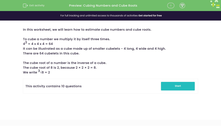# Cubing Numbers and Cube Roots

In this worksheet, students practise cubing numbers and working with cube roots.Key stage:  KS 3

Curriculum topic:   Number

Curriculum subtopic:   Understand Integer Powers/Real Roots

Difficulty level:#### Worksheet Overview

In this worksheet, we will learn how to estimate cube numbers and cube roots.

To cube a number we multiply it by itself three times.

43 = 4 x 4 x 4 = 64

It can be illustrated as a cube made up of smaller cubelets - 4 long, 4 wide and 4 high.

There are 64 cubelets in this cube.

The cube root of a number is the inverse of a cube.

The cube root of 8 is 2, because 2 × 2 × 2 = 8.

We write 3√8 = 2

### What is EdPlace?

We're your National Curriculum aligned online education content provider helping each child succeed in English, maths and science from year 1 to GCSE. With an EdPlace account you’ll be able to track and measure progress, helping each child achieve their best. We build confidence and attainment by personalising each child’s learning at a level that suits them.

Get started